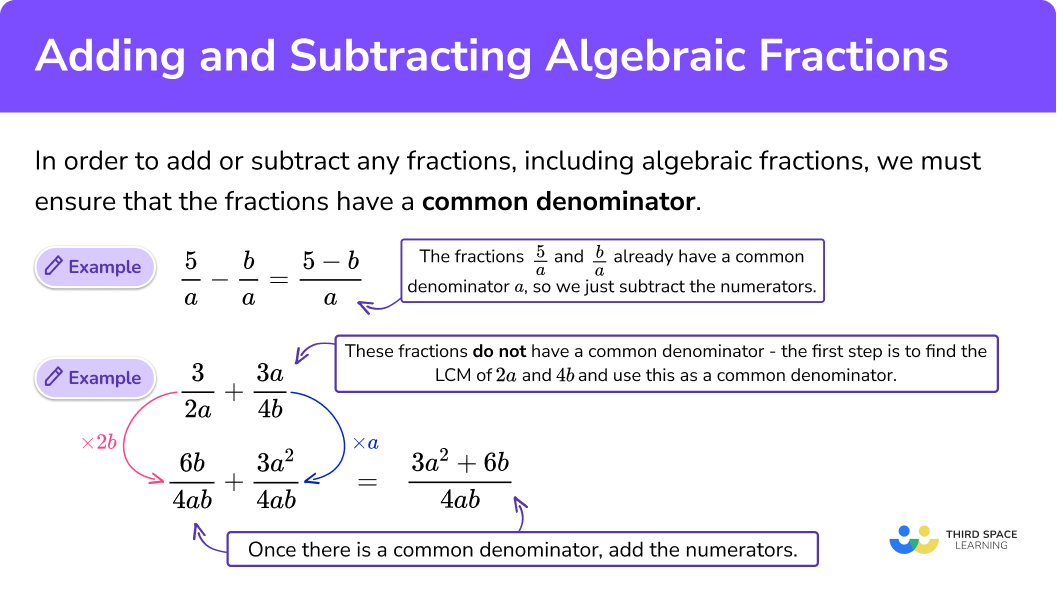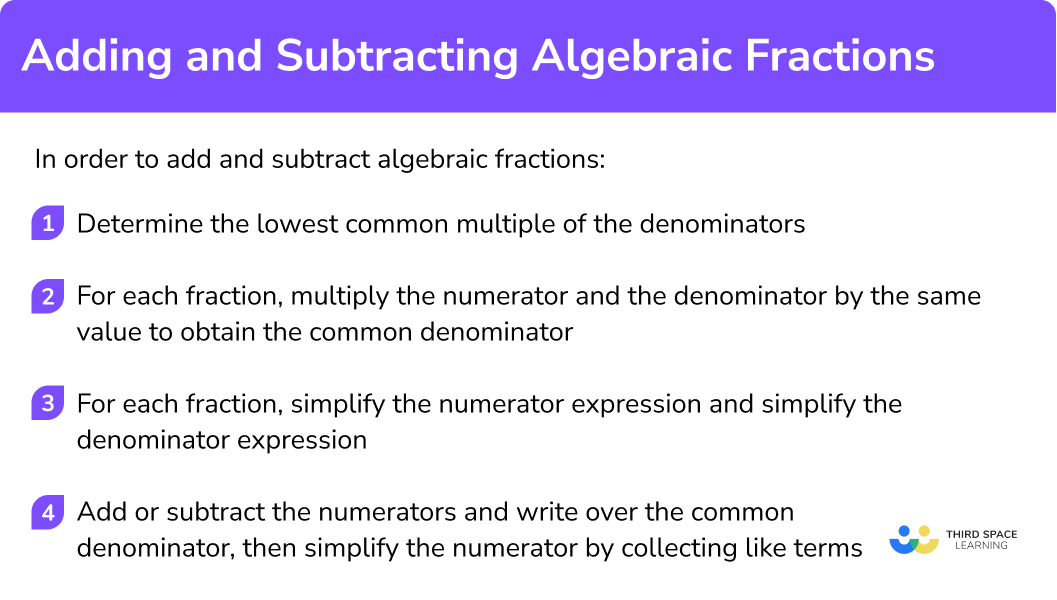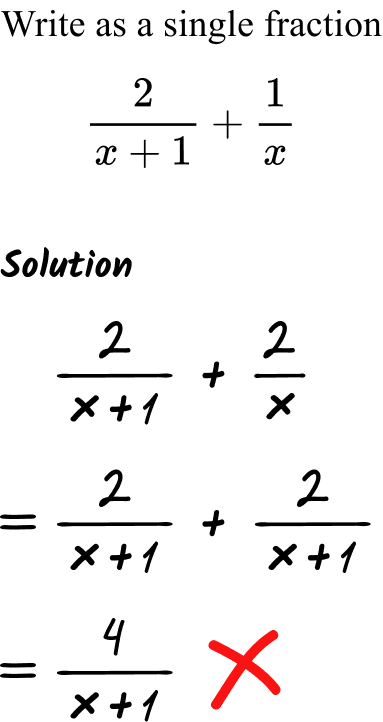# Adding And Subtracting Algebraic Fractions

Here we will learn about adding and subtracting algebraic fractions, including algebraic fractions with single and binomial denominators. We will also look at some problems involving quadratics and the difference of two squares.

There are also adding and subtracting algebraic fractions worksheets based on Edexcel, AQA and OCR exam questions, along with further guidance on where to go next if you’re still stuck.

## What is adding and subtracting algebraic fractions?

Adding and subtracting algebraic fractions is the skill of adding and subtracting two or more fractions that contain algebraic terms.

For example, \cfrac{3}{2x+3}, \, \cfrac{5}{y^{2}} \, , \, or \, \cfrac{2ab}{3a+4b} \, .

In order to add or subtract fractions we must ensure that the fractions have a common denominator.

For example, \cfrac{5}{2a^2} + \cfrac{c}{6ab} \, .

Finding the LCM of the algebraic denominators 2a^2 and 6ab using a Venn diagram.

2a^2 = 2\times a \times a

6ab = 2\times 3 \times a \times b

LCM = a \times 2\times a\times 3\times b =6a^{2}b

So the first denominator, 2a^2 , needs multiplying by 3b in order to make 6a^{2}b .

The second denominator, 6ab , needs multiplying by a in order to make 6a^{2}b .

Remember to multiply the numerator and denominator of a fraction by the same thing in order to write an equivalent fraction.

\cfrac{5\times 3b}{2a^2\times 3b} + \cfrac{c\times a}{6ab\times a} = \cfrac{15b}{6a^{2}b} + \cfrac{ac}{6a^{2}b} = \cfrac{15b+ac}{6a^{2}b}

Step-by-step guide: Adding and subtracting fractions

Step-by-step guide: Algebraic terms

### What is adding and subtracting algebraic fractions?### Fractions with a common denominator

If the fractions already have a common denominator then the numerators can be easily added/subtracted and the final answer will also have the same denominator. For algebraic fractions you may need to use additional skills such as simplifying expressions by collecting like terms.

For example,

\cfrac{5}{a}-\cfrac{b}{a} \, =\cfrac{5-b}{a}

\cfrac{2t}{k+1} \, +\cfrac{t}{k+1} \, =\cfrac{2t+t}{k+1} \, =\cfrac{3t}{k+1}

### Fractions without a common denominator

If the fractions have different denominators, we then need to find the lowest common multiple (LCM) of those denominators. Then we can use equivalent fractions to write all the fractions in the question with a common denominator.

Finding the LCM of numerical denominators will be a familiar skill. Additional skills such as expanding brackets and collecting like terms may be required for the algebraic numerators.

For example,

\cfrac{7a+3}{5}+\cfrac{4a-1}{10} \, .

The LCM of 5 and 10 is 10.

\cfrac{(7a+3)\times 2}{5\times 2} \, + \cfrac{4a-1}{10} \, =\cfrac{14a+6}{10} \, +\cfrac{4a-1}{10} \, =\cfrac{14a+6+4a-1}{10} \, = \cfrac{18a+5}{10}

### Finding the algebraic LCM

Finding the lowest common multiple of algebraic denominators can involve many different skills including identifying unique algebraic factors and factorising algebraic expressions.

Here are some examples of finding common algebraic denominators.

• Find the LCM of monomial expressions.
In order to work out the LCM of algebraic denominators with single terms (known as monomial expressions) you need to identify the common algebraic factors and the unique algebraic factors of each term.

You may also need to use the skill of finding the LCM of any numerical values involved. You then multiply together the
– common algebraic factors
– unique algebraic factors
– numerical LCM
to find the overall LCM for the algebraic terms.

For example,

• Using Venn diagrams to find the LCM
It can also be helpful to use Venn diagrams to find the LCM of algebraic terms. First you need to write the algebraic terms as products of their factors (using prime factors for the numerical part of the term).

Then you put the factors into a Venn diagram, and the LCM will be the result of all the factors in the Venn diagram multiplied together.

For example,

• Multiply the denominators together to a common denominator
Another technique to find a common denominator is to multiply the two denominators together.

This technique does not always find the lowest common multiple but does find a common multiple which can be used as the common denominator. This technique can be very useful when there are denominators with more than one term.

For example,

• LCM of binomial and quadratic expressions
Another technique for finding the LCM of algebraic denominators with more than one term is to factorise the expressions first. This can sometimes help to identify the LCM by highlighting common factors.

For example,

Step-by-step guide: Factorising

Step-by-step guide: Difference of two squares

## How to add and subtract algebraic fractions

In order to add and subtract algebraic fractions:

1. Determine the lowest common multiple of the denominators.
2. For each fraction, multiply the numerator and the denominator by the same value to obtain the common denominator.
3. For each fraction, simplify the numerator expression and simplify the denominator expression.
4. Add/subtract the numerators together and write over the common denominator.

### How to add and subtract algebraic fractions## Adding and subtracting algebraic fractions examples

### Example 1: adding fractions with monomial algebraic denominators

Write as a single fraction in the simplest form,

\cfrac{2}{a} \, +\cfrac{3}{b} \, .

1. Determine the lowest common multiple of the denominators.

Sometimes the lowest common multiple can be found by simply multiplying the two denominators together. This is the case here.

The lowest common multiple of a and b is a\times{b} which we can write as ab.

2For each fraction, multiply the numerator and the denominator by the same value to obtain the common denominator.

Multiply the numerator and the denominator of the first fraction by b.

Multiply the numerator and the denominator of the second fraction by a.

3For each fraction, simplify the numerator expression and simplify the denominator expression.

The numerator of the first fraction becomes 2\times{b}=2b.

The numerator of the second fraction becomes 3\times{a}=3a.

The common denominator is ab.

This gives us the equivalent calculation \cfrac{2b}{ab} \, +\cfrac{3a}{ab} \, .

4Add/subtract the numerators together and write over the common denominator.

\cfrac{2b}{ab} \, +\cfrac{3a}{ab} \, =\cfrac{2b+3a}{ab}

The numerator is 2b+3a.

This expression cannot be simplified because 2b and 3a are not like terms.

The final answer is \cfrac{2b+3a}{ab} \, .

### Example 2: subtracting fractions with monomial algebraic denominators

Write as a single fraction in the simplest form,

\cfrac{6}{e}\,-\cfrac{5}{f} \, .

Determine the lowest common multiple of the denominators.

For each fraction, multiply the numerator and the denominator by the same value to obtain the common denominator.

For each fraction, simplify the numerator expression and simplify the denominator expression.

Add/subtract the numerators together and write over the common denominator.

### Example 3: adding algebraic fractions with numerical denominators

Write as a single fraction in the simplest form,

\cfrac{3x}{4} \, +\cfrac{x}{6} \, .

Determine the lowest common multiple of the denominators.

For each fraction, multiply the numerator and the denominator by the same value to obtain the common denominator.

For each fraction, simplify the numerator expression and simplify the denominator expression.

Add/subtract the numerators together and write over the common denominator.

### Example 4: subtracting algebraic fractions with numerical numerators

Write as a single fraction in the simplest form,

\cfrac{5x}{7}\,-\cfrac{2x}{3} \, .

Determine the lowest common multiple of the denominators.

For each fraction, multiply the numerator and the denominator by the same value to obtain the common denominator.

For each fraction, simplify the numerator expression and simplify the denominator expression.

Add/subtract the numerators together and write over the common denominator.

### Example 5: adding algebraic fractions with numerical denominators

Write as a single fraction in the simplest form,

\cfrac{2x+1}{3} \, +\cfrac{x+2}{2} \, .

Determine the lowest common multiple of the denominators.

For each fraction, multiply the numerator and the denominator by the same value to obtain the common denominator.

For each fraction, simplify the numerator expression and simplify the denominator expression.

Add/subtract the numerators together and write over the common denominator. Simplify the numerator expression by collecting like terms.

### Example 6: subtracting algebraic fractions with numerical denominators

Write as a single fraction in the simplest form,

\cfrac{2x+1}{4} \, -\cfrac{x-1}{5} \, .

Determine the lowest common multiple of the denominators.

For each fraction, multiply the numerator and the denominator by the same value to obtain the common denominator.

For each fraction, simplify the numerator expression and simplify the denominator expression.

Add/subtract the numerators together and write over the common denominator. Simplify the numerator expression by collecting like terms.

### Example 7: adding algebraic fractions where one fraction has a binomial denominator

Write as a single fraction in the simplest form,

\cfrac{5}{x} \, +\cfrac{2}{x+1} \, .

Determine the lowest common multiple of the denominators.

For each fraction, multiply the numerator and the denominator by the same value to obtain the common denominator.

For each fraction, simplify the numerator expression and simplify the denominator expression.

Add/subtract the numerators together and write over the common denominator. Simplify the numerator expression by collecting like terms.

### Example 8: subtracting algebraic fractions where one fraction has a binomial denominator

Write as a single fraction in the simplest form,

\cfrac{3}{x+2} \, -\cfrac{6}{3x} \, .

Determine the lowest common multiple of the denominators.

For each fraction, multiply the numerator and the denominator by the same value to obtain the common denominator.

For each fraction, simplify the numerator expression and simplify the denominator expression.

Add/subtract the numerators together and write over the common denominator. Simplify the numerator expression by collecting like terms.

### Example 9: adding algebraic fractions where the denominators share a common binomial factor

Write as a single fraction in the simplest form,

\cfrac{5}{x+3}+\frac{1}{2x+6} \, .

Determine the lowest common multiple of the denominators.

For each fraction, multiply the numerator and the denominator by the same value to obtain the common denominator.

For each fraction, simplify the numerator expression and simplify the denominator expression.

Add/subtract the numerators together and write over the common denominator. Simplify the numerator expression by collecting like terms.

### Example 10: adding algebraic fractions where both fractions have a binomial denominator

Write as a single fraction in the simplest form,

\cfrac{5}{x+2} \, +\cfrac{3}{x-2} \, .

Determine the lowest common multiple of the denominators.

For each fraction, multiply the numerator and the denominator by the same value to obtain the common denominator.

For each fraction, simplify the numerator expression and simplify the denominator expression.

Add/subtract the numerators together and write over the common denominator.

### Example 11: subtracting algebraic fractions where both fractions have a binomial denominator

Write as a single fraction in the simplest form,

\cfrac{x}{x-2} \, -\cfrac{2x}{x+3} \, .

Determine the lowest common multiple of the denominators.

For each fraction, multiply the numerator and the denominator by the same value to obtain the common denominator.

For each fraction, simplify the numerator expression and simplify the denominator expression.

Add/subtract the numerators together and write over the common denominator. Simplify the numerator expression by collecting like terms.

### Example 12: adding algebraic fractions with binomial denominators including the difference of two squares

Write as a single fraction in the simplest form,

\cfrac{x+5}{x^{2}-1} \, +\cfrac{3x-2}{x+1} \, .

Determine the lowest common multiple of the denominators.

For each fraction, multiply the numerator and the denominator by the same value to obtain the common denominator.

For each fraction, simplify the numerator expression and simplify the denominator expression.

Add/Subtract the numerators together and write over the common denominator. Simplify the numerator expression by collecting like terms.

## Common misconceptions

• Not recognising double negatives when subtracting algebraic fractions
Some students fail to recognise the double negative when subtracting fractions in a question such as this.

\cfrac{2x}{7} \, -\cfrac{x-1}{7}

For example a student may incorrectly write the following working out.

In these steps the student has failed to subtract both of the terms of the numerator of the second fraction. To avoid this mistake it is advisable to write the numerators in brackets, as this helps to remind you that all the terms in the bracket need to be subtracted. The correct solution looks like this.

\cfrac{2x}{7}\,-\cfrac{x-1}{7}= \cfrac{(2x)-(x-1)}{7} \, = \cfrac{2x-x+1}{7} \, =\cfrac{x+1}{7}

• Adding or subtracting a value to the numerator and the denominator of a fraction in an attempt to write an equivalent fraction

To write an equivalent fraction we must multiply or divide both the numerator and denominator by the same value. Sometimes students incorrectly assume that they can do the same with addition or subtraction. For example, a student may incorrectly write the following working out.

The fraction \cfrac{3}{x} \, is not equivalent to \cfrac{3+1}{x+1} \, =\cfrac{4}{x+1} \, .

To avoid this mistake you must remember that you can only multiply or divide the numerator and denominator by the same value to write an equivalent fraction.

The lowest common multiple of the denominators in this question can be found by multiplying the denominators together (x)\times(x+1) = x(x+1). The correct solution looks like this.

## Practice adding and subtracting algebraic fractions questions

1. Write as a single fraction in the simplest form,

\cfrac{1}{c} \, +\cfrac{4}{d} \, .

\cfrac{5}{c+d}\cfrac{d+4}{c+d}\cfrac{5}{cd}\cfrac{4c+d}{cd}\begin{aligned} &\cfrac{1}{c} \, +\cfrac{4}{d}\\\\ &=\cfrac{1\times{d}}{c\times{d}} \, +\cfrac{4\times{c}}{d\times{c}}\\\\ &=\cfrac{d}{cd}\, +\cfrac{4c}{cd}\\\\ &=\cfrac{d+4c}{cd}\\\\ &=\cfrac{4c+d}{cd} \end{aligned}

2. Write as a single fraction in the simplest form,

\cfrac{9}{g} \, -\cfrac{7}{h} \, .

\cfrac{9g-7h}{gh}\cfrac{2}{g-h}\cfrac{9h-7g}{gh}\cfrac{2}{gh}\begin{aligned} &\cfrac{9}{g} \, -\frac{7}{h}\\\\ &=\cfrac{9\times{h}}{g\times{h}} \, -\cfrac{7\times{g}}{h\times{g}}\\\\ &=\cfrac{9h}{gh} \, -\cfrac{7g}{gh}\\\\ &=\cfrac{9h-7g}{gh} \end{aligned}

3. Write as a single fraction in the simplest form,

\cfrac{2x}{3} \, +\cfrac{5x}{6} \, .

\cfrac{3x}{2}\cfrac{7x}{9}\cfrac{x}{2}\cfrac{7x^{2}}{9}\begin{aligned} &\cfrac{2x}{3} \, +\cfrac{5x}{6} \\\\ &=\cfrac{2x\times{2}}{3\times{2}} \, +\cfrac{5x}{6}\\\\ &=\cfrac{4x}{6} \, +\cfrac{5x}{6}\\\\ &=\cfrac{9x}{6} \end{aligned}

4. Write as a single fraction in the simplest form,

\cfrac{4x}{7} \, -\cfrac{2x}{5} \, .

\cfrac{6x}{35}x\cfrac{18x}{35}3x\begin{aligned} &\cfrac{4x}{7} \, -\cfrac{2x}{5}\\\\ &=\cfrac{4x\times{5}}{7\times{5}} \, -\cfrac{2x\times{7}}{5\times{7}}\\\\ &=\cfrac{20x}{35} \, -\cfrac{14x}{35}\\\\ &=\cfrac{20x-14x}{35}\\\\ &=\cfrac{6x}{35} \end{aligned}

5. Write as a single fraction in the simplest form,

\cfrac{3x+5}{4} \, +\cfrac{x+1}{2} \, .

3x\cfrac{5x+7}{4}\cfrac{2x+3}{3}\cfrac{5x+6}{4}\begin{aligned} &\cfrac{3x+5}{4} \, +\cfrac{x+1}{2}\\\\ &=\cfrac{3x+5}{4} \, +\cfrac{(x+1)\times{2}}{2\times{2}}\\\\ &=\cfrac{3x+5}{4}\, +\cfrac{2x+2}{4}\\\\ &=\cfrac{3x+5+2x+2}{4}\\\\ &=\cfrac{5x+7}{4} \end{aligned}

6. Write as a single fraction in the simplest form,

\cfrac{3x+2}{2} \, -\cfrac{x-1}{4} \, .

x+15x+3\cfrac{5(x+1)}{4}\cfrac{5x+3}{4}\begin{aligned} &\cfrac{3x+2}{2} \, -\cfrac{x-1}{4}\\\\ &=\cfrac{(3x+2)\times{2}}{2\times{2}} \, -\cfrac{x-1}{4}\\\\ &=\cfrac{6x+4}{4} \, -\cfrac{x-1}{4}\\\\ &=\cfrac{6x+4-(x-1)}{4}\\\\ &=\cfrac{6x+4-x+1}{4}\\\\ &=\cfrac{5x+5}{4}\\\\ &=\cfrac{5(x+1)}{4} \end{aligned}

7. Write as a single fraction in the simplest form,

\cfrac{1}{2x} \, +\cfrac{4}{x+2} \, .

\cfrac{5}{3x+2}\cfrac{11x}{2x(x+2)}\cfrac{5}{2x(x+2)}\cfrac{9x+2}{2x(x+2)}\begin{aligned} &\cfrac{1}{2x} \, +\cfrac{4}{x+2}\\\\ &=\cfrac{1\times{(x+2)}}{2x\times{(x+2)}} \, +\cfrac{4\times{2x}}{(x+2)\times{2x}}\\\\ &=\cfrac{x+2}{2x(x+2)} \, +\cfrac{8x}{2x(x+2)}\\\\ &=\cfrac{x+2+8x}{2x(x+2)}\\\\ &=\cfrac{9x+2}{2x(x+2)} \end{aligned}

8. Write as a single fraction in the simplest form,

\cfrac{3}{x+4} \, -\cfrac{5}{2x} \, .

\cfrac{x+20}{2x(x+4)}\cfrac{x-20}{2x(x+4)}\cfrac{2}{x-4}\cfrac{x+4}{2x(x+4)}\begin{aligned} &\cfrac{3}{x+4} \, -\cfrac{5}{2x}\\\\ &=\cfrac{3\times{2x}}{(x+4)\times{2x}} \, -\cfrac{5\times{(x+4)}}{2x\times{(x+4)}}\\\\ &=\cfrac{6x}{2x(x+4)} \, -\cfrac{5x+20}{2x(x+4)}\\\\ &=\cfrac{6x-(5x+20)}{2x(x+4)}\\\\ &=\cfrac{6x-5x-20)}{2x(x+4)}\\\\ &=\cfrac{x-20}{2x(x+4)} \end{aligned}

9. Write as a single fraction in the simplest form,

\cfrac{4}{3(x-1)} \, -\cfrac{1}{x-1} \, .

\cfrac{1}{3(x-1)}\cfrac{1}{x-1}\cfrac{3}{2(x-1)}\cfrac{1}{3x-1}\begin{aligned} &\cfrac{4}{3(x-1)} \, -\cfrac{1}{x-1}\\\\ &=\cfrac{4}{3(x-1)} \, -\cfrac{1\times{3}}{(x-1)\times{3}}\\\\ &=\cfrac{4}{3(x-1)} \, -\cfrac{3}{3(x-1)}\\\\ &=\cfrac{4-3}{3(x-1)}\\\\ &=\cfrac{1}{3(x-1)} \end{aligned}

10. Write as a single fraction in the simplest form,

\cfrac{10}{x-3} \, -\cfrac{4}{x-1} \, .

\cfrac{2(3x+1)}{(x-3)(x-1)}-3\cfrac{2(3x+1)}{(x-3)(x-1)}\cfrac{8x}{(x-3)(x-1)}\begin{aligned} &\cfrac{10}{x-3} \, -\cfrac{4}{x-1}\\\\ &=\cfrac{10\times{(x-1)}}{(x-3)\times{(x-1)}} \, -\cfrac{4\times{(x-3)}}{(x-1)\times{(x-3)}}\\\\ &=\cfrac{10x-10}{(x-3)(x-1)} \, -\cfrac{4x-12}{(x-3)(x-1)}\\\\ &=\cfrac{10x-10-(4x-12)}{(x-3)(x-1)}\\\\ &=\cfrac{10x-10-4x+12}{(x-3)(x-1)}\\\\ &=\cfrac{6x+2}{(x-3)(x-1)}\\\\ &=\cfrac{2(3x+1)}{(x-3)(x-1)} \end{aligned}

11. Write as a single fraction in the simplest form,

\cfrac{3x}{x+5} \, +\cfrac{x}{x+1} \, .

\cfrac{4x(x+2)}{(x+5)(x+1)}\cfrac{4x}{(x+5)(x+1)}\cfrac{2x(x+2)}{x+3}\cfrac{3x^{2}}{2(x+3)}\begin{aligned} &\cfrac{3x}{x+5} \, +\cfrac{x}{x+1}\\\\ &=\cfrac{3x\times{(x+1)}}{(x+5)\times(x+1)} \, +\cfrac{x\times{(x+5)}}{(x+1)\times{(x+5)}}\\\\ &=\cfrac{3x^{2}+3x}{(x+5)(x+1)} \, +\cfrac{x^{2}+5x}{(x+5)(x+1)}\\\\ &=\cfrac{3x^{2}+3x+x^{2}+5x}{(x+5)(x+1)}\\\\ &=\cfrac{4x^{2}+8x}{(x+5)(x+1)}\\\\ &=\cfrac{4x(x+2)}{(x+5)(x+1)} \end{aligned}

12. Write as a single fraction in the simplest form,

\cfrac{x-1}{x+2} \, -\cfrac{x-1}{x^{2}-4} \, .

\cfrac{x^{2}-1}{x^{2}-4}\cfrac{(x-5)(x-1)}{(x+2)(x-2)}\cfrac{(x-3)(x-1)}{(x+2)(x-2)}x-2\begin{aligned} &\cfrac{x-1}{x+2} \, -\cfrac{x-1}{x^{2}-4}\\\\ &=\cfrac{(x-1)\times{(x-2)}}{(x+2)\times{(x-2)}} \, -\cfrac{x-1}{(x+2)(x-2)}\\\\ &=\cfrac{(x-1)(x-2)}{(x+2)(x-2)} \, -\cfrac{x-1}{(x+2)(x-2)}\\\\ &=\cfrac{x^{2}-3x+2}{(x+2)(x-2)} \, -\cfrac{x-1}{(x+2)(x-2)}\\\\ &=\cfrac{x^{2}-3x+2-(x-1)}{(x+2)(x-2)}\\\\ &=\cfrac{x^{2}-3x+2-x+1}{(x+2)(x-2)}\\\\ &=\cfrac{x^{2}-4x+3}{(x+2)(x-2)}\\\\ &=\cfrac{(x-3)(x-1)}{(x+2)(x-2)} \end{aligned}

## Adding and subtracting algebraic fractions GCSE questions

1.  Write as a single fraction, \cfrac{1}{x} \, +\cfrac{1}{y} \, -\cfrac{1}{z} \, .

(2 marks)

\cfrac{yz}{xyz} \, +\cfrac{xz}{xyz} \, -\cfrac{xy}{xyz}

(1)

\cfrac{yz+xz-xy}{xyz}

(1)

Alternative method 1

\cfrac{y+x}{xy} \, -\cfrac{1}{z}

(1)

\cfrac{z(y+x)-xy}{xyz} \; or \, \cfrac{yz+xz-xy}{xyz}

(1)

Alternative method 2

\cfrac{1}{x} \, +\cfrac{z-y}{yz}

(1)

\cfrac{yz+x(z-y)}{xyz} \; or \, \cfrac{yz+xz-xy}{xyz}

(1)

Alternative method 3

\cfrac{1}{y} \, +\cfrac{z-x}{xz}

(1)

\cfrac{xz+y(z-x)}{xyz} \; or \cfrac{xz+yz-xy}{xyz}

(1)

2.  Lucia is answering the question,(a)  Describe the mistake that Lucia has made in her working out.

(b)  Determine the correct solution to \cfrac{2}{x+1} \, +\cfrac{1}{x} \, .

(4 marks)

(a) She has added 1 to the numerator and denominator of the second fraction in an attempt to make a common denominator of x+1.

However \cfrac{2}{x+1} is not equivalent to \cfrac{1}{x} \, .

(1)

(b)

\cfrac{2\times{x}}{x(x+1)} \, +\cfrac{1\times{(x+1)}}{x(x+1)}

(1)

\cfrac{2x+x+1}{x(x+1)}

(1)

\cfrac{3x+1}{x(x+1)}

(1)

3. Write 2-x+\cfrac{x^{2}-4}{x+4} \, as a single fraction of the form \cfrac{a(b-x)}{x+c} \,  where a, b, and c are integers.

(4 marks)

\cfrac{(2-x)(x+4)}{x+4} \, +\cfrac{x^{2}-4}{x+4}

(1)

\cfrac{2x+8-x^{2}-4x}{x+4} \, +\cfrac{x^{2}-4}{x+4}

(1)

\cfrac{4-2x}{x+4}

(1)

\cfrac{2(2-x)}{x+4} or a=2, b=2, c=4

(1)

## Learning checklist

You have now learned how to:

•  Add and subtract algebraic fractions

## Still stuck?

Prepare your KS4 students for maths GCSEs success with Third Space Learning. Weekly online one to one GCSE maths revision lessons delivered by expert maths tutors.

Find out more about our GCSE maths tuition programme.